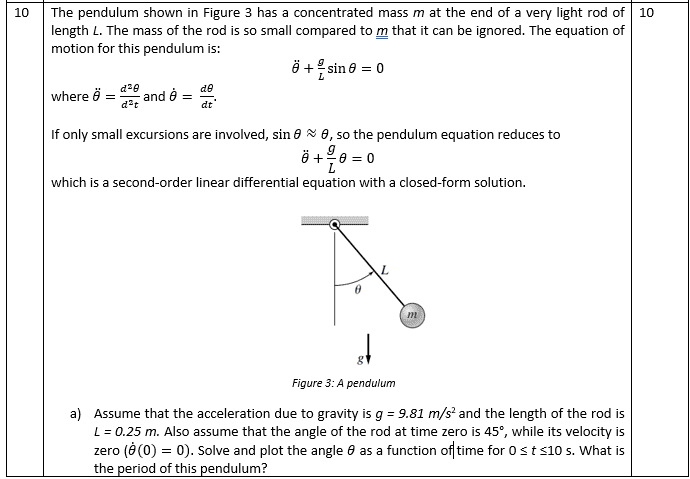# Pendulum Question (Matlab Coursework)

some bloke
Homework Statement:
The pendulum shown in Figure 3 has a concentrated mass m at the end of a very light rod of length L. The mass of the rod is so small compared to m that it can be ignored. The equation of motion for this pendulum is:
θ ̈+g/L sin⁡θ=0
where θ ̈=(d^2 θ)/(d^2 t) and θ ̇= dθ/dt.

If only small excursions are involved, sin⁡θ≈θ, so the pendulum equation reduces to
θ ̈+g/L θ=0
which is a second-order linear differential equation with a closed-form solution.

Assume that the acceleration due to gravity is g = 9.81 m/s2 and the length of the rod is L = 0.25 m. Also assume that the angle of the rod at time zero is 45°, while its velocity is zero (θ ̇(0)=0). Solve and plot the angle θ as a function of time for 0 ≤ t ≤10 s. What is the period of this pendulum?
What happens if the initial conditions are changed? Experiment with a 10°, then a 145° starting angle of the rod, considering initial velocity zero in both cases. Compare the period of oscillation with the theoretical period (hint: the angular frequency ω=√(g/L) ).
Suppose that the pendulum were moved to the top pf a mountain where the gravitation acceleration decreases to g = 9.6 m/s2. What would happen to the period of oscillation of the pendulum?
Relevant Equations:
θ ̈+g/L sin⁡θ=0, θ ̈+g/L θ=0
Apologies for my lack of knowledge on the equations front, I have burnt by brain out on this and haven't the capacity to learn LaTex right now! So here's a screengrab of it:So, This is a Matlab coursework and I am struggling to work out how best to approach solving it. What I have so far is that:
(with D2 as the second Derivative and D as the first derivative)

D2=-g*sin(theta)/L, as given
D= integral of this, which is g*cos(theta)/L, and this is the angular velocity.
Therefore D = (981*cos(theta))/25)+C (simplified by Matlab into integers)

The question provides that at T=0, Theta = 45 and D=0, so I can say that C = -981*cos(45)/25.
I also know that at T=?, Theta = 0 and D is at it's maximum.

What I cannot fathom is how to get Theta as a function of Time out of this. I know that D is the angular velocity, so change in displacement over time, and I know that D2 is the angular accelleration, which is the change of velocity over time. I know that there's a way to do this, but I cannot dig far enough into my A-level maths of 9 years ago to find it. Helpfully, I've been advised that I will be taught how to do these things next year, after the coursework has been graded. Helpful.

Coding it into Matlab I can do, but I don't know what I'm coding. So, my biggest question is this: How to I get this strange equation of F''(theta)+f(theta)=0 to somehow give an answer in terms of t? Where does "t" come into it?

Homework Helper
Gold Member
2022 Award
I'm unclear what the question is asking you to do.
The exact equation, with the sin θ, has no solution in closed form. That is why the approximation for small oscillations is generally used. That can be solved, as it notes.
But then it gives an initial angle of 45°, definitely not small, and says "solve".
Either they do not expect you to solve the differential equation, just use Matlab to plot it, or they want you to solve the approximation and use that as though 45° is small enough.

some bloke
Exactly! This is where I'm getting confused! and the next section asks to sub in 145 degrees!
I think I've muddled my way into getting Matlab to plot it. Looks like the period is around 8 seconds, or just under. Going to get it to regugitate a number for me shortly!

Homework Helper
Gold Member
2022 Award
Exactly! This is where I'm getting confused! and the next section asks to sub in 145 degrees!
I think I've muddled my way into getting Matlab to plot it. Looks like the period is around 8 seconds, or just under. Going to get it to regugitate a number for me shortly!
Can you solve the approximate form of the ODE?

Gold Member
Coding it into Matlab I can do, but I don't know what I'm coding. So, my biggest question is this: How to I get this strange equation of F''(theta)+f(theta)=0 to somehow give an answer in terms of t? Where does "t" come into it?
I would think you are to solve the motion of the pendulum using the small angle formula with MathLab.
Though it does not state explicitly.
Maybe you are to compare the two equations and the deviation of the exact to the small angle.

Note that it gives you the angular frequency of the pendulum from
Compare the period of oscillation with the theoretical period (hint: the angular frequency ω=√(g/L) ).
do you know how to get the period from that equation.
Somehow an 8 sec second period seems a bit long.

I did find this.
https://www.mathworks.com/help/symbolic/simulate-physics-pendulum-swing.html
Maybe it is what you need to solve and play around with the program.